# 薄板分析的线性基梯度光滑伽辽金无网格法1)

(厦门大学土木工程系，厦门 361005);(厦门市交通基础设施智能管养工程技术研究中心，厦门 361005)

# A GRADIENT SMOOTHING GALERKIN MESHFREE METHOD FOR THIN PLATE ANALYSIS WITH LINEAR BASIS FUNCTION1)

Deng Like, Wang Dongdong2), Wang Jiarui, Wu Junchao

Department of Civil Engineering, Xiamen University, Xiamen 361005, China；Xiamen Engineering Technology Center for Intelligent Maintenance of Infrastructures, Xiamen 361005, China

Abstract

The fourth order governing equation of thin plate necessitates the employment of C$^{1}$ continuous shape functions with a minimum degree of two in a Galerkin formulation. Thus at least a quadratic basis function should be utilized in meshfree approximation to enable the Galerkin meshfree thin plate analysis. However, due to the rational nature of reproducing kernel meshfree shape functions, the computation of the second order derivatives of meshfree shape functions is quite complex and costly, which also requires expensive high order Gauss quadrature rules to properly integrate the stiffness matrix. In this work, a gradient smoothing Galerkin meshfree method with particular reference to the linear basis function is proposed for thin plate analysis. The foundation of the present development is the construction of smoothed meshfree gradients with linear basis function, where the second order smoothed gradients are expressed as combinations of standard first order gradients and the computational burden is remarkably reduced. Furthermore, it is shown that the smoothed meshfree gradients with linear basis function satisfy both the linear and quadratic gradient consistency conditions and consequently they are adequate for thin plate analysis in the context of Galerkin formulation. An interpolation error study is given as well to validate the higher order consistency conditions and applicability of smoothed meshfree gradients for Galerkin analysis of thin plates. It turns out that efficient lower order Gauss integration rules now work well for the proposed method. Numerical results demonstrate that compared with the conventional Galerkin meshfree method with quadratic basis function, the proposed gradient smoothing Galerkin meshfree method with linear basis function yields similar convergence rates, but with better accuracy and less integration points for stiffness computation.

Keywords： Galerkin meshfree method ; linear basis function ; thin plate ; gradient smoothing ; consistency condition

0

Deng Like, Wang Dongdong, Wang Jiarui, Wu Junchao. A GRADIENT SMOOTHING GALERKIN MESHFREE METHOD FOR THIN PLATE ANALYSIS WITH LINEAR BASIS FUNCTION1)[J]. Chinese Journal of Theoretical and Applied Mechanics, 2019, 51(3): 690-702 https://doi.org/10.6052/0459-1879-19-004

## 1 薄板控制方程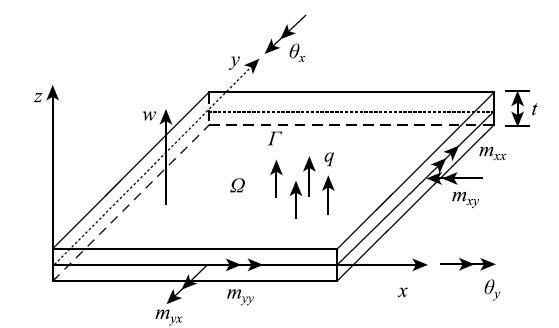Fig. 1   Sign convention of thin plate

$${\theta} = \left\{ {\begin{matrix} {\theta _x } \\ {\theta _y } \\ \end{matrix} } \right\} = \left\{ {{\begin{matrix} {w_{,x} } \\ {w_{,y} } \\ \end{matrix} }} \right\}\tag{1}$$

$$\kappa = \left\{ {\begin{matrix} {\kappa _{xx} } \\ {\kappa _{yy} } \\ {2\kappa _{xy} } \\ \end{matrix} } \right\} = \left\{ {\begin{matrix} {w_{,xx} } \\ {w_{,yy} } \\ {2w_{,xy}} \\ \end{matrix} } \right\}\tag{2}$$

\begin{equation}\label{eq3} { { m}} = - { { D}} \kappa\tag{3}\end{equation}

$${ { m}} = \mbox{\{}m_{xx} \mbox{ }m_{yy} \mbox{ }m_{xy} \mbox{\}}^{\rm T}\tag{4}$$

$${ { D}} = \bar {D}\left[ {{\begin{matrix}{*{20}c} 1&amp; \nu&amp; 0\\ \nu&amp; 1&amp; 0\\ 0&amp; 0&amp; {(1 - \nu ) / 2}\\ \end{matrix} }} \right],\mbox{ }\bar {D} = \frac{Et^3}{12(1 - \nu ^2)}\tag{5}$$

$$\int_\varOmega {\delta \kappa ^{\rm T}{ { m}}{\rm d}\varOmega } + \int_\varOmega {\delta wq{\rm d}\varOmega } + \\ \qquad \int_{\varGamma ^w} {\delta \lambda ^w(w - \bar {w}){\rm d}\varGamma } + \int_{\varGamma ^w} {\lambda ^w\delta w{\rm d}\varGamma } + \\ \qquad \int_{\varGamma ^\theta } {\delta \lambda ^\theta (\theta _n-\bar {\theta }_n )} {\rm d}\varGamma + \int_{\varGamma ^\theta } {\lambda ^\theta \delta \theta _n } {\rm d}\varGamma = 0 \tag{6}$$

## 2 无网格形函数

$$\varphi _s (r) = \\ \dfrac{1}{5!}\left\{\!\! {{\begin{array}{*{20}l} {(3 \!-\! 3r)^5 \!-\! 6(2 \!-\! 3r)^5 \!+\! 15(1 \!-\! 3r)^5}, {r \le \dfrac{\mbox{1}}{3}} \\ {(3 - 3r)^5 - 6(2 - 3r)^5}, \ \ \ {\dfrac{\mbox{1}}{3}< r \le \dfrac{2}{3}} \\ {(3 - 3r)^5} ,\ \ \ \ {\dfrac{2}{3} < r \le 1} \\ 0, \ \ \ \ {r > 1} \\ \end{array} }} \right.\\ \tag{10}$$

\begin{equation} \label{eq12} \sum\limits_{I = 1}^{NP} {\varPsi _I ({ { x}}){ {p}}\mbox{(}{ { x}}_I )} = { { p}}({ { x}})\tag{12} \end{equation}

\begin{equation} \label{eq13} \sum\limits_{I = 1}^{NP} {\varPsi _I ({ { x}}){ {p}}\mbox{(}{ { x}}_I - { { x}})} = { { p}}({\bf{ 0}})\tag{13} \end{equation}

\begin{equation} \label{eq14} { { A}}({ { x}}){ { c}}({ { x}}) = { { p}}({ { x}})\tag{14} \end{equation}

\begin{equation} \label{eq15} { { A}}({ { x}}) = \sum\limits_{I = 1}^{NP} {{ {p}}({ { x}}_I - { { x}}){ { p}}^{\rm T}({ { x}}_I-{ {x}})\phi _s ({ { x}}_I - { { x}})}\tag{15} \end{equation}

\begin{equation} \label{eq16} \varPsi _I ({ { x}}) = { { p}}^{\rm T}( 0){ { A}}^{ - 1}({ { x}}){ { p}}({ { x}}_I - { { x}})\varphi _s ({ { x}}_I - { { x}})\tag{16} \end{equation}

$$\varPsi _{I,i} ({ { x}}) = { { p}}^{\rm T}( 0)\{{ { A}}_{,i}^{ - 1} { { p}}_I \phi _{sI} + { { A}}^{ - 1}{ { p}}_{I,i} \phi _{sI} + \\ { { A}}^{ - 1}{ { p}}_I \phi _{sI,i} \}\tag{17}$$

$$\varPsi _{I,ij} ({ { x}}) = { { p}}^{\rm T}( 0)\{{ { A}}_{,ij}^{ - 1} { { p}}_I \phi _{sI} + { { A}}_{,i}^{ - 1} { { p}}_{I,j} \phi _{sI} + \\ { { A}}_{,i}^{ - 1} { { p}}_I \phi _{sI,j} \mbox{ + }{ { A}}_{,j}^{ - 1} { { p}}_{I,i} \phi _{sI} + { { A}}^{ - 1}{ { p}}_{I,ij} \phi _{sI}+ \\ { { A}}^{ - 1}{ { p}}_{I,i} \phi _{sI,j} + { { A}}_{,j}^{ - 1} { { p}}_I \phi _{sI,i} \mbox{ + }{ { A}}^{ - 1}{ { p}}_{I,j} \phi _{sI,i}+ \\ { { A}}^{ - 1}{ { p}}_I \phi _{sI,ij} \} \tag{18}$$

$$\left. \begin{array}{l} { {A}}_{,i}^{ - 1} = - {{ {A}}^{-1}}{{ {A}}_{,i}}{{ {A}}^{ - 1}}\\[3mm] { {A}}_{,ij}^{ - 1} = - {{ {A}}^{ - 1}}({{ {A}}_{,ij}}{{ {A}}^{ - 1}} + {{ {A}}_{,i}}{ {A}}_{,j}^{ - 1} + {{ {A}}_{,j}}{ {A}}_{,i}^{ - 1}) \end{array} \right\}\tag{19}$$

\begin{equation} \label{eq20} \left. {\begin{array}{l} \sum\limits_{I = 1}^{NP} {\varPsi _{I,x} ({ { x}})y_I } = \sum\limits_{I = 1}^{NP} {\varPsi _{I,y} ({ { x}})x_I } = 0 \\[5mm] \sum\limits_{I = 1}^{NP} {\varPsi _{I,x} ({ { x}})x_I } = \sum\limits_{I = 1}^{NP} {\varPsi _{I,y} ({ { x}})y_I } = 1 \\ \end{array}} \right\}\tag{20} \end{equation} \begin{equation} \label{eq21} \left. {\begin{array}{l} \sum\limits_{I = 1}^{NP} {\varPsi _{I,xx} ({ { x}})x_I^2 } = \sum\limits_{I = 1}^{NP} {\varPsi _{I,yy} ({ { x}})y_I^2 } = 2 \\[5mm] \sum\limits_{I = 1}^{NP} {\varPsi _{I,xy} ({ { x}})x_I y_I } = 1 \\ \end{array}} \right\}\tag{21} \end{equation}

## 3 无网格梯度光滑方法及一致性条件

### 3.1 无网格形函数的光滑梯度

\begin{equation} \label{eq22} \tilde {\varPsi }_{I,i} ({ { x}}) = \int_\varOmega \vartheta ({ { x}},{ { y}})\varPsi _{I,i} ({ { y}}){\rm d}\varOmega\tag{22} \end{equation}

$$\bar {\varPsi }_{I,i} ({ { x}}) = \sum\limits_{J = 1}^{NP} {\vartheta ({ { x}},{ { x}}_J )\varPsi _{I,i} ({ { x}}_J )} = \\ \sum\limits_{J = 1}^{NP} \varPsi _J ({ { x}})\varPsi _{I,i} ({ { x}}_J )\tag{23}$$

$$\label{eq24} \bar {\varPsi }_{I,ij}({ { x}}) = \sum\limits_{J = 1}^{NP} \varPsi _{J,j} ({ { x}})\varPsi _{I,i} ({ { x}}_J )\tag{24}$$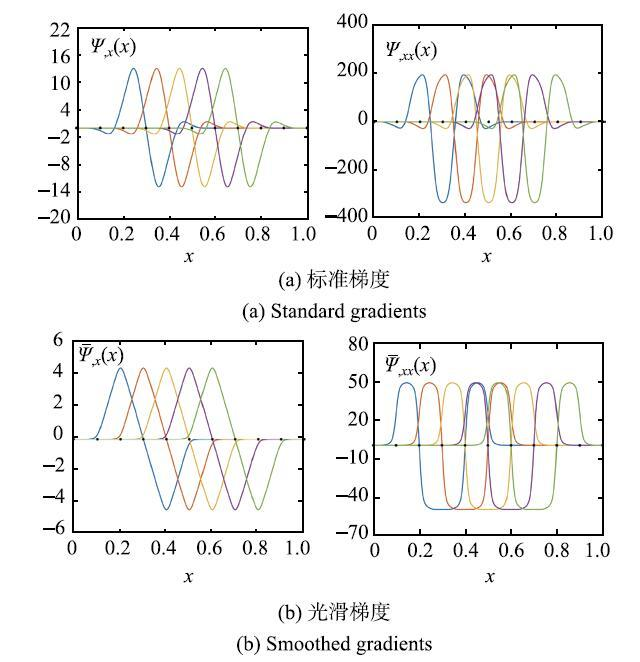Fig. 2   Comparison of 1D standard and smoothed meshfree gradients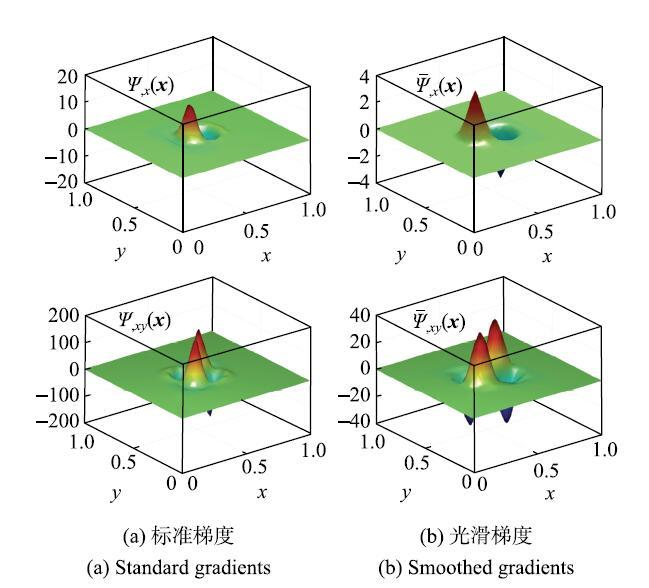Fig. 3   Comparison of 2D standard and smoothed meshfree gradients

### 3.2 线性基形函数光滑梯度的线性一致性条件

$$\sum\limits_{I = 1}^{NP} {\bar {\varPsi }_{I,y} ({ { x}})x_I } = \sum\limits_{I = 1}^{NP} {\sum\limits_{J = 1}^{NP} {\varPsi _J ({ {x}})\varPsi _{I,y} ({ { x}}_J )x_I } } =\\ \sum\limits_{J = 1}^{NP} {\varPsi _J ({ { x}})\underbrace {\sum\limits_{I = 1}^{NP} {\varPsi _{I,y} ({ { x}}_J )x_I } }_{ = 0}} = 0 \tag{25}$$

$$\sum\limits_{I = 1}^{NP} {\bar {\varPsi }_{I,x} ({ { x}})x_I } = \sum\limits_{I = 1}^{NP} {\sum\limits_{J = 1}^{NP} {\varPsi _J ({ {x}})\varPsi _{I,x} ({ { x}}_J )x_I } } = \\ \sum\limits_{J = 1}^{NP} {\varPsi _J ({ { x}})\underbrace {\sum\limits_{I = 1}^{NP} {\varPsi _{I,x} ({ { x}}_J )x_I } }_{ = 1}} =\\ \sum\limits_{J = 1}^{NP} {\varPsi _J ({ { x}})} = 1\tag{26}$$

\begin{equation} \label{eq27} \sum\limits_{I = 1}^{NP} {\bar {\varPsi }_{I,x} ({ {x}})y_I } = 0,\mbox{ }\sum\limits_{I = 1}^{NP} {\bar {\varPsi }_{I,y} ({ { x}})y_I } = 1\tag{27} \end{equation}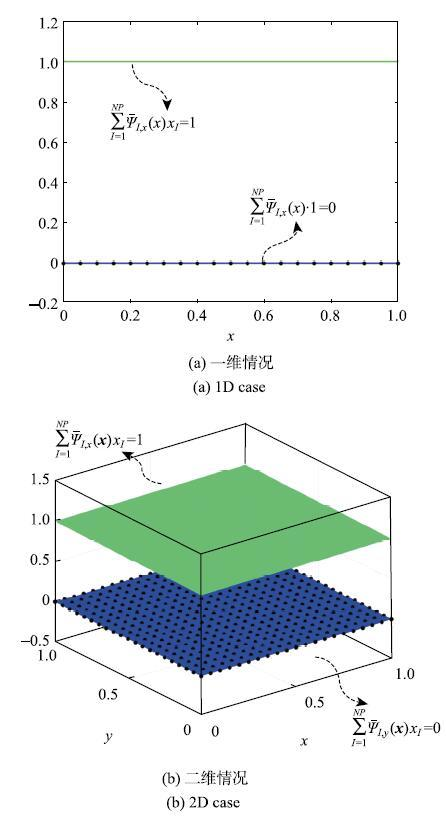Fig. 4   Standard consistency conditions for the first order smoothed meshfree gradients with linear basis function

### 3.3 线性基形函数光滑梯度的二阶一致性条件

$$\sum\limits_{I = 1}^{NP} {\bar {\varPsi }_{I,xx} ({ { x}})x_I^2 } = \sum\limits_{J = 1}^{NP} {\varPsi _{J,x} ({ { x}})} \sum\limits_{I = 1}^{NP} {\varPsi _{I,x} ({ { x}}_J )(x_I - x_J + x_J )^2}= \\ \sum\limits_{J = 1}^{NP} {\varPsi _{J,x} ({ { x}})x_J^2 } \underbrace{\sum\limits_{I = 1}^{NP} \varPsi _{I,x} ({ { x}}_J )} _{ = 0} +\\ 2\sum\limits_{J = 1}^{NP} \varPsi _{J,x} ({ { x}})x_J \underbrace {\sum\limits_{I = 1}^{NP} \varPsi _{I,x} ({ { x}}_J )x_{IJ}} _{ = 1}+ \\ \sum\limits_{J = 1}^{NP} {\varPsi _{J,x} ({ { x}})} \sum\limits_{I = 1}^{NP} {\varPsi _{I,x} ({ { x}}_J )} x_{IJ}^2 =\\ 2\underbrace{\sum\limits_{J = 1}^{NP} {\varPsi _{J,x} ({ { x}})x_J } }_{ = 1} +\\ \sum\limits_{J = 1}^{NP} {\varPsi _{J,x} ({ { x}})} \sum\limits_{I = 1}^{NP} {\varPsi _{I,x} ({ { x}}_J )} x_{IJ}^2= \\ 2 + \sum\limits_{J = 1}^{NP} {\varPsi _{J,x} ({ { x}})} \sum\limits_{I = 1}^{NP} {\varPsi _{I,x} ({ { x}}_J )} x_{IJ}^2 \tag{28}$$

\begin{equation} \label{eq29} \sum\limits_{I = 1}^{NP} {\varPsi _{I,x} ({ { x}}_J )} x_{IJ}^2 = \sum\limits_{K = 1}^{NP} {\varPsi _{K,x} ({ { x}}_L )} x_{KL}^2\tag{29} \end{equation}

\begin{equation} \label{eq30} \sum\limits_{I = 1}^{NP} {\bar {\varPsi }_{I,xx} ({ {x}})x_I^2 } = 2 + \sum\limits_{K = 1}^{NP} {\varPsi _{K,x} ({ {x}}_L )} x_{KL}^2 \underbrace {\sum\limits_{J = 1}^{NP} {\varPsi _{J,x} ({ { x}})} }_{ = 0} = 2\tag{30} \end{equation}

\begin{equation} \label{eq31} \sum\limits_{I = 1}^{NP} {\bar {\varPsi }_{I,xx} ({ {x}})x_I^2 } = 2\tag{31} \end{equation}

$$\mathop{\sum}\limits_{I = 1}^{NP} \bar{\varPsi }_{I,yy} ({ {x}})y_I^2 = 2\tag{32}$$

$$\mathop{\sum}\limits_{I = 1}^{NP} \bar{\varPsi }_{I,xy} ({ {x}})x_I y_I = 1\tag{33}$$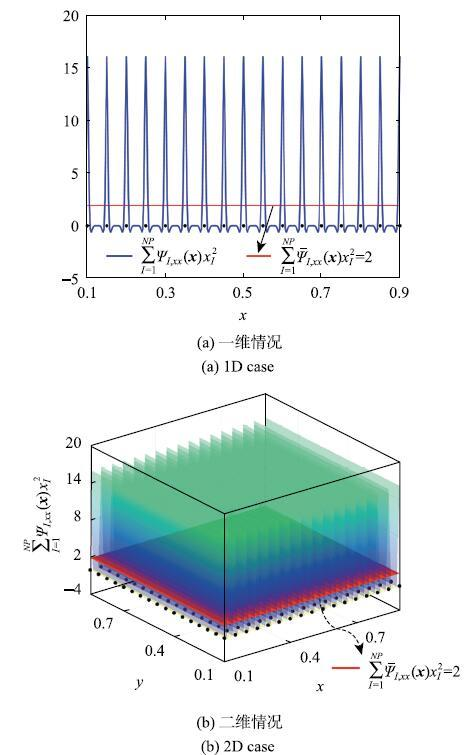Fig. 5   Extra quadratic consistency conditions for the second order smoothed meshfree gradients with linear basis function

### 3.4 插值误差分析

$$L_2 \mbox{error = }\bigg(\int_\varOmega {(w - w^h)^2{\rm d}\varOmega \bigg)^{1 / 2}} \bigg/ \bigg(\int_\varOmega {w^2{\rm d}\varOmega } \bigg)^{1 / 2}\tag{34}$$

$$H_{s_1 }\mbox{error} = \bigg(\int_\varOmega {(w_{,i} - w_{,i}^h )^2{\rm d}\varOmega } \bigg)^{1 / 2} \bigg/ \bigg(\int_\varOmega {w_{,i}^2 {\rm d}\varOmega } \bigg)^{1 / 2}\tag{35}$$

$$H_{s_2 } \mbox{error} = \bigg(\int_\varOmega {(w_{,ij} - w_{,ij}^h )^2{\rm d}\varOmega } \bigg)^{1 / 2} \bigg/ \bigg(\int_\varOmega {w_{,ij}^2 {\rm d}\varOmega } \bigg)^{1 / 2}\tag{36}$$

$$w(x) = {\rm e}^x + \frac{1}{6}(1 - {\rm e})x^3 - \frac{1}{2}x^2 + \left(\frac{4}{3} - \frac{5}{6}{\rm e}\right)x - 1\tag{37}$$

$$w({ { x}}) = \sin (\pi x)\sin (\pi y)\tag{38}$$

\begin{equation} \label{eq39} w_{,i}^h ({ { x}}) = \sum\limits_{I\mbox{ = }1}^{NP} {\bar {\varPsi }_{I,i} ({ { x}})d_I }\tag{39} \end{equation} \begin{equation} \label{eq40} w_{,ij}^h ({ { x}}) = \sum\limits_{I\mbox{ = }1}^{NP} {\bar {\varPsi }_{I,ij} ({ { x}})d_I }\tag{40} \end{equation}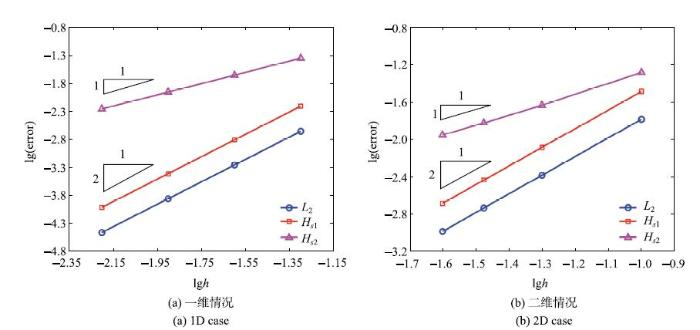Fig. 6   Comparison of interpolation errors of smoothed meshfree gradients with linear basis function

## 4 薄板分析的线性基梯度光滑伽辽金无网格法离散方程

\begin{equation}\label{eq41} {{\bar{ K}\bar {d}}} = {{\bar{ f}}}\tag{41} \end{equation}

$${{ \bar { K}}}\mbox{ = }\left[ {{\begin{array}{*{20}c} { {K}}& {{ { G}}^{w{\rm T}} }& {{ { G}}^{\theta {\rm T}} }\\ {{ { G}}^w }& {\bf{ 0}}& {\bf{ 0}}\\ {{ { G}}^\theta }& {\bf{ 0}}& {\bf{ 0}} \\ \end{array} }} \right]\tag{42}$$

$${{ \bar { d}}} = \left\{ {{\begin{array}{*{20}c} { { d}}\\ { { \lambda }}^w \\ { { \lambda }}^\theta \\ \end{array} }} \right\}\mbox{, }{{ \bar { f}}} = \left\{ {{\begin{array}{*{20}c} { { f}}\\ { { q}}^w \\ { { q}}^\theta \\ \end{array} }} \right\}\tag{43}$$

$${ {K}} = \mathop {\rm A}\limits_{I,J = 1}^{NP} [{K_{IJ}}{\rm{], }}~{ {G}}^w = \mathop {\rm A}\limits_{I,K = 1}^{NP,NW} [G_{IK}^w{\rm{]}},{\rm{ }}{ {G}}_{}^\theta = \mathop {\rm A}\limits_{I,L = 1}^{NP,NR} [G_{IL}^\theta {\rm{]}}\qquad \tag{44}$$

$${ {d}} = \mathop {\rm A}\limits_{I = 1}^{NP} [{d_I}{\rm{]}},~{\rm{ }}{{ {\lambda }}^w} = \mathop {\rm A}\limits_{K = 1}^{NW} [\lambda _K^w{\rm{], }}~{{ {\lambda }}^\theta } = \mathop {\rm A}\limits_{L = 1}^{NR} [\lambda _L^\theta {\rm{]}}\tag{45}$$

$${ {f}} = \mathop {\rm A}\limits_{I = 1}^{NP} [{f_I}{\rm{]}},~{\rm{ }}{{ {q}}^w} = \mathop {\rm A}\limits_{K = 1}^{NW} [q_K^w{\rm{], }}~{{ {q}}^\theta } = \mathop {\rm A}\limits_{L = 1}^{NR} [q_L^\theta {\rm{]}}\tag{46}$$

$$K_{IJ} \mbox{ = }\int_\varOmega {{{ \bar { B}}}_I^{\rm T} {{ D\bar { B}}}_J {\rm d}\varOmega } ,\mbox{ }{{ \bar { B}}}_I = \left\{ {{\begin{array}{*{20}c} {\bar {\varPsi }_{I,xx} }\\ {\bar {\varPsi }_{I,yy} }\\ {\bar {\varPsi }_{I,xy} + \bar {\varPsi }_{I,yx} }\\ \end{array} }} \right\}\tag{47}$$

$$G_{IK}^w = - \int_{\varGamma ^w} {\varPsi _I N_K } {\rm d}\varGamma ,\mbox{ }G_{IL}^\theta = - \int_{\varGamma ^\theta } {\bar {\varPsi }_{I,n} N_L } {\rm d}\varGamma\tag{48}$$

$$f_I = \int_\varOmega {\varPsi _I q{\rm d}\varOmega }\tag{49}$$

$$q_K^w = - \int_{\varGamma ^w} {N_K \bar {w}{\rm d}\varGamma } \mbox{, }q_L^\theta = - \int_{\varGamma ^\theta } {N_L \bar {\theta }_n {\rm d}\varGamma }\tag{50}$$

## 5 算例

### 5.1 欧拉梁问题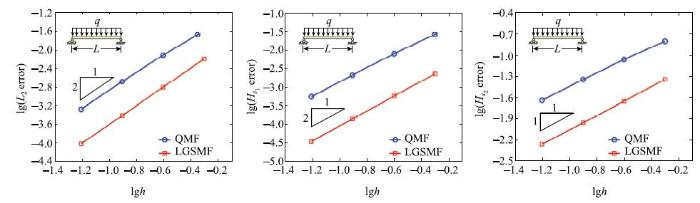Fig. 7   Convergence comparison for the simply supported beam problem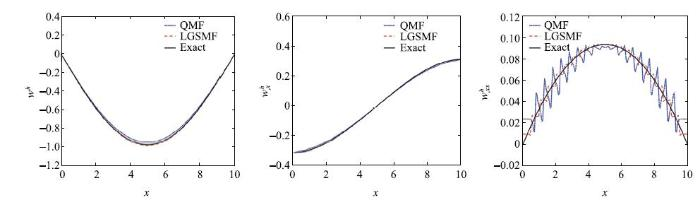Fig. 8   Comparison of results for the simply supported beam problem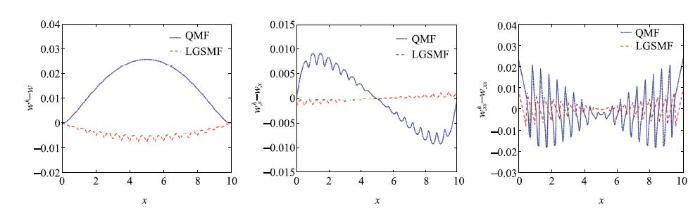Fig. 9   Error comparison for the simply supported beam problem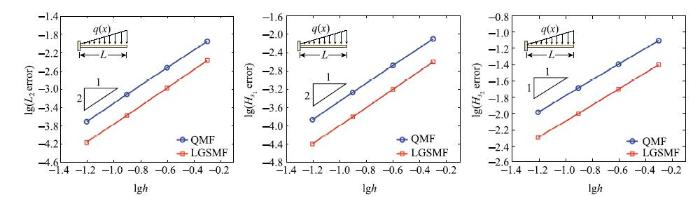Fig. 10   Convergence comparison for the cantilever beam problem

### 5.2 简支方板和矩形板问题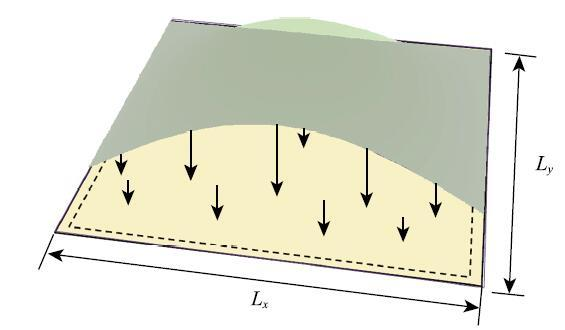Fig. 11   Description of the simply supported square plate problem

\begin{equation} \label{eq51} w({ { x}}) = - \sin \left(\frac{\pi x}{L_x }\right)\sin \left(\frac{\pi y}{L_y }\right)\tag{51} \end{equation}

$$q({ x})=-\bar{D}\left(\dfrac{\pi^2}{L^2_x}+\dfrac{\pi^2}{L^2_y}\right)^2\sin \left(\dfrac{\pi x}{L_x}\right)\sin\left(\dfrac{\pi y}{L_y}\right)$$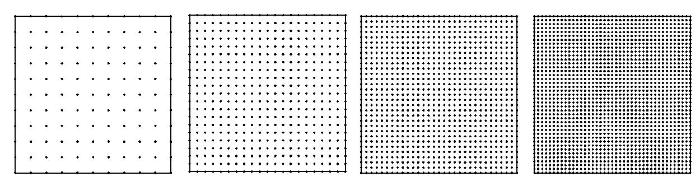Fig. 12   Meshfree discretizations for the simply supported square plate problem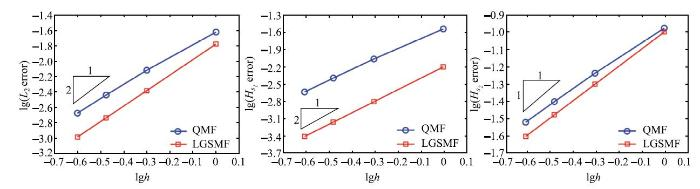Fig. 13   Convergence comparison for the simply supported square plate problem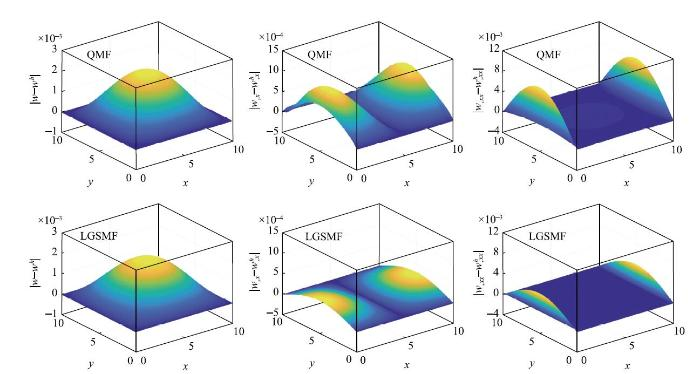Fig. 14   Error comparison for the simply supported square plate problem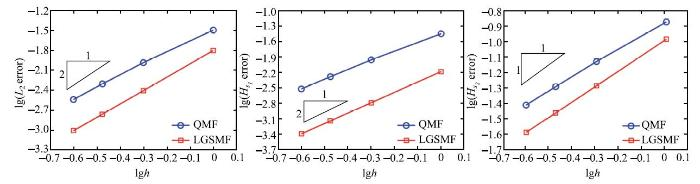Fig. 15   Convergence comparison for the simply supported rectangular plate problem

### 5.3 固支圆板问题Fig. 16   Description of the clamped circular plate problem

\begin{equation} \label{eq52} w({ { x}}) = \frac{q}{64\bar {D}}(R^2 - x^2-y^2)^2\tag{52} \end{equation}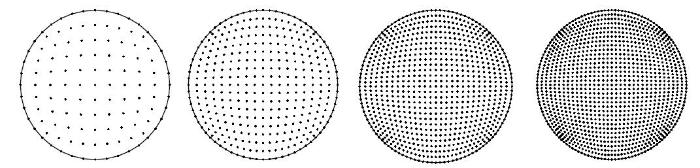Fig. 17   Meshfree discretizations for the clamped circular plate problem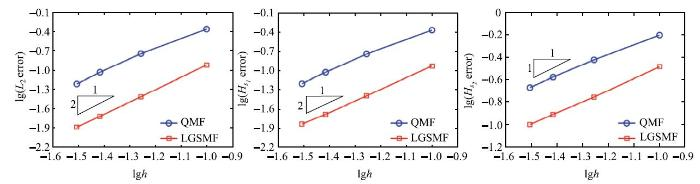Fig. 18   Convergence comparison for the clamped circular plate problem

## 6 结论

The authors have declared that no competing interests exist.

## 参考文献 原文顺序 文献年度倒序 文中引用次数倒序 被引期刊影响因子〈〉